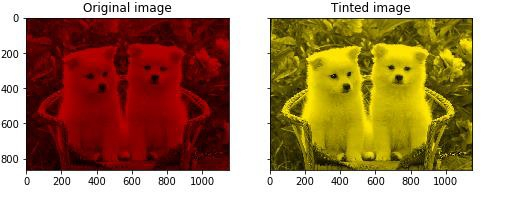# How can a specific tint be added to grayscale images in scikit-learn in Python?

PythonServer Side ProgrammingProgramming

The values of ‘R’, ‘G’, and ‘B’ are changed and applied to the original image to get the required tint.

Below is a Python program that uses scikit-learn to implement the same. Scikit-learn, commonly known as sklearn is a library in Python that is used for the purpose of implementing machine learning algorithms −

## Example

import matplotlib.pyplot as plt
from skimage import data
from skimage import color
path = "path to puppy_1.jpg"
grayscale_img = rgb2gray(orig_img)
image = color.gray2rgb(grayscale_img)
red_multiplier = [0.7, 0, 0]
yellow_multiplier = [1, 0.9, 0]
fig, (ax1, ax2) = plt.subplots(ncols=2, figsize=(8, 4),
sharex=True, sharey=True)
ax1.imshow(red_multiplier * image)
ax1.set_title('Original image')
ax2.imshow(yellow_multiplier * image)
ax2.set_title('Tinted image')

## Output## Explanation

The required packages are imported into the environment.

• The path where the image is stored is defined.

• The ‘imread’ function is used to visit the path and read the image.

• The ‘imshow’ function is used to display the image on the console.

• The function ‘rgb2gray’ is used to convert the image from RGB color space to grayscale color space.

• The function ‘gray2rgb’ is used to convert the image from grayscale to RGB color space.

• The matplotlib library is used to plot this data on console.

• The R, G, B values for the multipliers are defined and applied on the image.

• Output is displayed on the console.